# SAT Math Multiple Choice Question 209: Answer and Explanation

### Test Information

Question: 209

14. A 40-foot tall arch with a parabolic shape has a line drawn between the bases of the two legs of the arch. If the height above the ground, y, of the arch can be written as the function y(x) = a(x - 20)(x + 20), where x represents the horizontal distance along the line between the bases from a point on the ground directly under the highest point of the arch, then what is the value of negative constant a ?

• A. -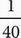• B. -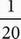• C. -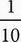• D. -20

C When the quadratic is set to 0 the parabola crosses the x-axis at (-20, 0) and (20, 0). Because parabolas are symmetrical, the vertex of the parabola is at (0, 40). Plug this point into the equation to get 40 = a(0 - 20)(0 + 20). Simplify the right side of the equation to get 40 = a(-20)(20) or 40 = -400a. Solve for a to get a = -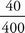= -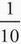. Therefore, the correct answer is (C).